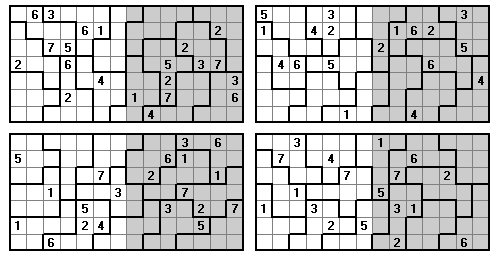Two Square Sudoku ProblemsThese problems are based on various sets of polyominoes arranged into an n x 2n rectangle. Each square contains the nu,bers 1 to n in each row and column and each polyomino has numbers 1 to n.

Double-six Sudoku

The basis of these problems is patterns created from the twelve piece set we get by adding two monominoes to a 2x2 square.Double-Seven Sudoku

It is difficult to find a complete set of 14 pieces from the heptominoes with any simple conditions. The following problems are from a set made by adding a domino to the P pentomino and removing concave pieces and those pieces with width two.Another type of two-square problem this time with a rectangle made with multiple copies of two heptominoes.Double-Eight Sudoku

These problems are based on constructions made from the set of 16 convex pieces we get if we add two squares to a 2x3 rectangle.Alternatively, we can use the set of convex pieces obtained by adding two parallel dominoes to a 2x2 square.